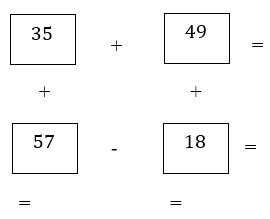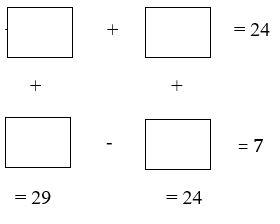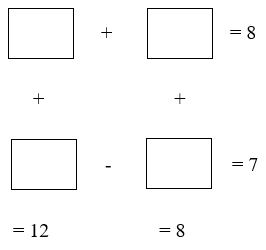Below are three addition- subtraction problems that are classified into Level 1, Level 2, and Level 3.

Level 1:    Find the sums and difference of the numbers indicated on the diagram (vertically/horizontally)Level 2:  Fill in the empty boxes such that the sums (difference) of the horizontally and vertically arranged numbers satisfy the given outputsLevel 3:   Fill in the empty boxes such that the sums (difference) of the horizontally and vertically arranged numbers satisfy the given outputs